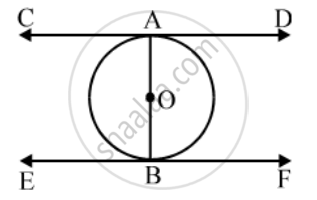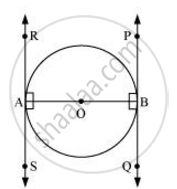# Prove that the Tangents Drawn at the Ends of a Diameter of a Circle Are Parallel. - Mathematics

Prove that the tangents drawn at the ends of a diameter of a circle are parallel.

Prove that tangents drawn at the ends of a diameter of a circle are parallel to each other.

#### Solution 1

Given: CD and EF are the tangents at the end points A and B of the diameter AB of a circle with centre O.To prove: CD || EF.

Proof: CD is the tangent to the circle at the point A.

EF is the tangent to the circle at the point B.

∴ ∠ABE = 90°

Thus, ∠BAD = ∠ABE (each equal to 90°).

But these are alternate interior angles.

∴ CD || EF

#### Solution 2Let AB be a diameter of the circle. Two tangents PQ and RS are drawn at points A and B respectively.

Radius drawn to these tangents will be perpendicular to the tangents.

Thus, OA ⊥ RS and OB ⊥ PQ

∠OAR = 90º

∠OAS = 90º

∠OBP = 90º

∠OBQ = 90º

It can be observed that

∠OAR = ∠OBQ (Alternate interior angles)

∠OAS = ∠OBP (Alternate interior angles)

Since alternate interior angles are equal, lines PQ and RS will be parallel.

Concept: Number of Tangents from a Point on a Circle
Is there an error in this question or solution?
Chapter 10: Circles - Exercise 10.2 [Page 214]

Share